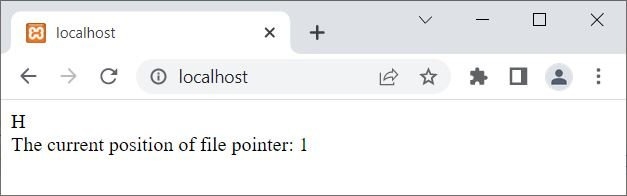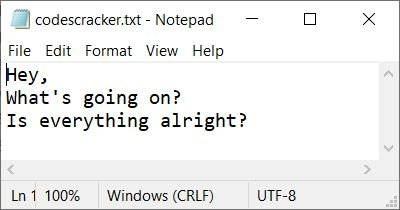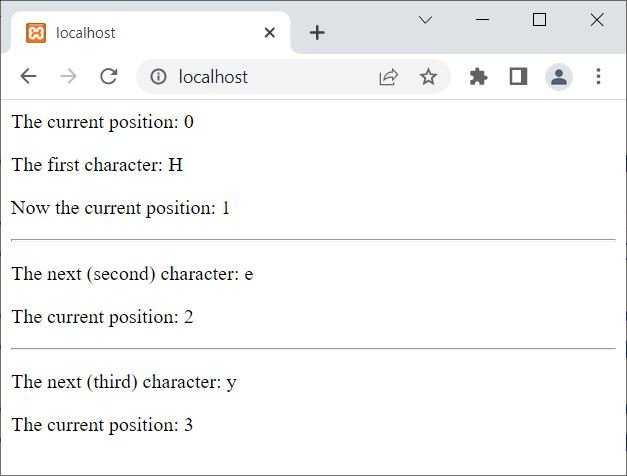# PHP ftell(): Find Current Position of File Pointer

The PHP ftell() function is used when we need to find the current position of the file pointer in the file. For example:

```<?php
\$file = "codescracker.txt";
\$fp = fopen(\$file, "r");

\$character = fgetc(\$fp);
echo \$character;
\$currentPos = ftell(\$fp);
echo "<br>The current position of file pointer: " . \$currentPos;

fclose(\$fp);
?>```

The output produced by the above PHP example on the ftell() function is:And here is the snapshot of the file codescracker.txt used in the above example:Let me modify the above example to convert to a self-defined example of the ftell() function in PHP:

```<?php
\$fp = fopen("codescracker.txt", "r");

if(\$fp)
{
echo "<p>The current position: " .ftell(\$fp). "</p>";

echo "<p>The first character: " .fgetc(\$fp). "</p>";
echo "<p>Now the current position: " .ftell(\$fp). "</p><hr>";

echo "<p>The next (second) character: " .fgetc(\$fp). "</p>";
echo "<p>The current position: " .ftell(\$fp). "</p><hr>";

echo "<p>The next (third) character: " .fgetc(\$fp). "</p>";
echo "<p>The current position: " .ftell(\$fp). "</p>";

fclose(\$fp);
}
else
echo "<p>Unable to open the file</p>";
?>```

Now the output should be:## PHP ftell() Syntax

The syntax of the ftell() function in PHP is:

`ftell(filePointer)`

### Advantages of the ftell() function in PHP

• Position of the file pointer is accurate thanks to the "ftell()" function, which is available for any given file.
• Useful for both reading and writing files: This function is helpful for a variety of file handling tasks because it can be used for both reading and writing files.
• Can be used with other file functions: To carry out more complex file operations, the "ftell()" function can be combined with other file functions like "fseek()" and "rewind()."

### Disadvantages of the ftell() function in PHP

• Not helpful for very large files: The "ftell()" function may not be helpful for very large files because it may return a value that is larger than the integer range.
• The "ftell()" function might not function properly with non-seekable streams, such as pipes or network sockets.
• Not thread-safe: The "ftell()" function is not thread-safe, which means that the outcome may be unpredictable if multiple threads access the same file.

PHP Online Test

« Previous Tutorial Next Tutorial »